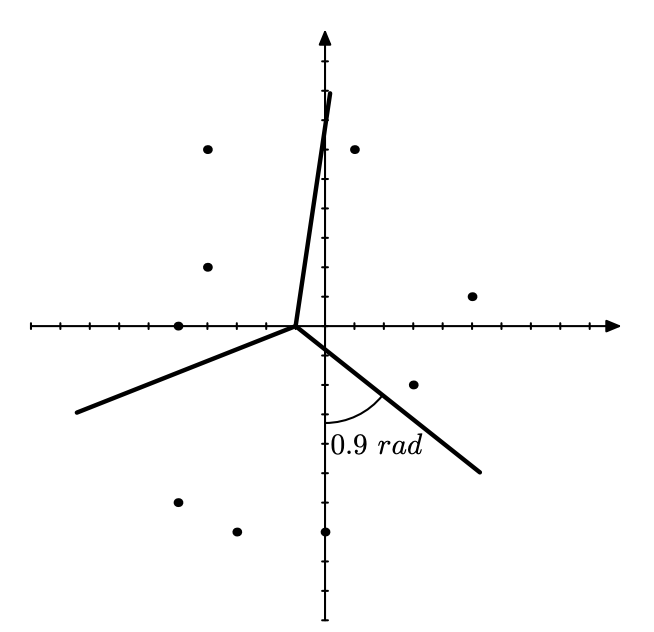시간 제한 메모리 제한 제출 정답 맞은 사람 정답 비율
3 초 512 MB 31 7 6 20.690%

## 문제

Serious debates often arise around chocolate cakes with cherries. Everyone likes cherries, so the cake must be cut in such a way that everyone receives a piece with the same number of cherries.

Two people can easily divide a cake with one straight cut using a simple knife. Though less obvious, two straight cuts are always enough for four people. But what if you want to cut a cake for three people? We have a solution!

You are given a cake with cherries and a Y-shaped knife. Cut the cake info three parts with equal number of cherries or report that it is impossible.

Formally, you are given a set of points in a plane in general position. A Y-shaped knife is an apex point and three infinite straight rays going from the apex. The angle between any two rays equals 120(2π/3). The knife divides the plane into three sections. You have to find the position of the apex and the rotation of the first ray such that each of the sections contains equal number of points.

## 입력

In the first line of input there is a single integer n, the number of points (1 ≤ n ≤ 105). Next n lines contain two integers each, x and y coordinates of the corresponding point. Coordinates do not exceed 106 by absolute value.

It is guaranteed that no two points coincide and no three points lie on the same line.

## 출력

If it is impossible to make the desired cut, print “No” (without quotes).

If it is possible, print “Yes” (without quotes). Then print three real numbers x, y, and r (|x|, |y| ≤ 107, 0 ≤ r < 2π/3), where x and y denote the coordinates of the apex and r denotes the counter-clockwise rotation of the knife in radians.

The initial position of the knife (that is, corresponding to zero rotation) is when one of the rays points down. The position when the ray points to the right corresponds to the rotation of π/2.

See “Notes” section for detailed explanation of the checker.

## 예제 입력 1

9
3 -2
-4 6
0 -7
-5 -6
5 1
1 6
-5 0
-3 -7
-4 2


## 예제 출력 1

Yes
-1 0 0.9## 힌트

All calculations are done in long double, an 80-bit floating-point type. Let the apex point be (x, y) with rotation r. For each input point (xi, yi):

• if |x − xi| < 10−9 and |y − yi| < 10−9, the solution gets “Wrong Answer”;
• the angle of the direction from the apex point to the input point is calculated as atan2(yi − y, xi − x) + π/2 − r modulo 2π, where atan2(y, x) is the polar angle of point (x, y);
• if the absolute difference of the angle and 0, 2π/3, or 4π/3 is less than 10−9, the solution gets “Wrong answer”;
• finally, the section which the point belongs to is calculated straightforwardly.